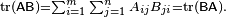## Eigen vector, Eigen value 3x3 Matrix Calculator

 Regular Matrix A =
 Scalar Matrix (Z=c×I)
|A| =
Singular Matrix (A - c×I) =
|A - c×I| =
Trace of A =
Eigenvalue of c3 =
 + i
Eigenvalue of c2 =
 + i
Eigenvalue of c1 =
 + i
c3 in Eigenvector (x,y,z) values =
c2 in Eigenvector (x,y,z) values =
c1 in Eigenvector (x,y,z) values =

An eigenvector of a square matrix A is a non-zero vector v that, when the matrix is multiplied by v, yields a constant multiple of v, the multiplier being commonly denoted by λ. That is: A v = λ v

(Because this equation uses post-multiplication by v, it describes a right eigenvector.) The number λ is called the eigenvalue of A corresponding to v.

All eigenvalues and eigenvectors satisfy the equation Ax = λx for a given square matrix A.

Simple matrix calculator to calculate the eigen value and eigen vector of a 3x3 matrix. Enter the values of the 3x3 matrix and click on the calculate button.

### Trace

The trace, tr(A) of a square matrix A is the sum of its diagonal entries. While matrix multiplication is not commutative as mentioned above, the trace of the product of two matrices is independent of the order of the factors:

tr(AB) = tr(BA).

This is immediate from the definition of matrix multiplication:Also, the trace of a matrix is equal to that of its transpose, i.e.,

tr(A) = tr(AT).

Thinkcalculator.com provides you helpful and handy calculator resources.